### Home > INT3 > Chapter 8 > Lesson 8.1.1 > Problem8-10

8-10.
1. A table is a useful tool for writing some inverse functions. When the function has only one x in its equation, the function can be described with a sequence of operations, each applied to the previous result. Consider the following table for f(x) = 2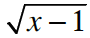+ 3. Homework Help ✎

2.  1st 2nd 3rd 4th What f does to x: subtracts 1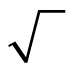multiplies by 2 adds 3
3. Since the inverse must undo these operations, in the opposite order, the table for f –1(x) would look like the one below.

4.  1st 2nd 3rd 4th What f –1 does to x: subtracts 3 divides by 2 ( )2 adds 1
1. Copy and complete the following table for g–1(x) if g(x) =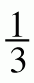(x + 1)2 – 2.

 1st 2nd 3rd 4th What g does to x: adds 1 ( )2 divides by 3 subtracts 2 What g–1 does to x:2. Write the equations for f –1(x) and g–1(x).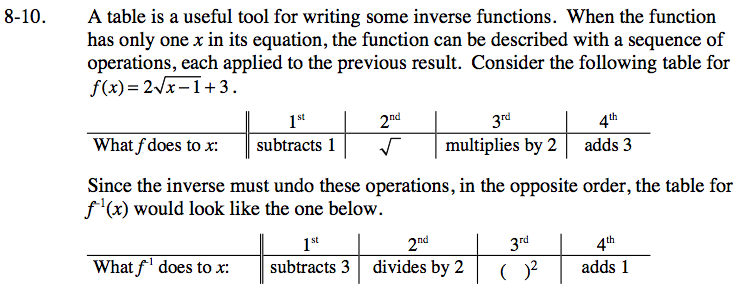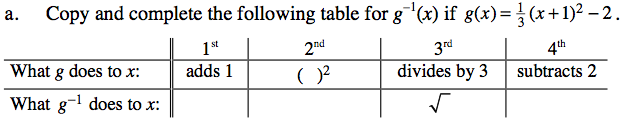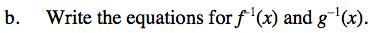$f^{-1}(x)=\left(\frac{x-3}{2}\right)^2+1$